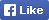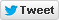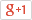Computer/Electronics Questions and Answers
C, C++, VC++, COM/DCOM, DLL and moreLoginSign upHome Electronics Computer Questions Member's Area News Site Map About

Write a program to find the solutions of the given quadratic equation.

Source Code

 1 #include 2 #include 3 #include 4 int main (int argc, char *argv[]) 5 { 6 clrscr(); 7 float a,b,c,r1,r2,t; 8 printf("\n Enter the co ordinates of the quadratic equation:"); 9 printf("\n Enter the value preceding x2: "); 10 scanf("%f",&a); 11 printf("\n Enter the value preceding x: "); 12 scanf("%f",&b); 13 printf("\n Enter the numeric value: "); 14 scanf("%f",&c); 15 t=(b*b)-(4*a*c); 16 printf("\n The roots of the quadratic equation %fx2+%fx+%f are:",a,b,c); 17 if(t==0) 18 { 19 r1=(b*-1)/(2*a); 20 printf("\n only one root : %f",r1); 21 } 22 else if(t<0) 23 { 24 printf(" imaginary."); 25 } 26 else 27 { 28 r1=((b*-1)+sqrt(t))/(2*a); 29 r2=((b*-1)-sqrt(t))/(2*a); 30 printf(" %f and %f",r1,r2); 31 } 32 getch(); 33 }

Find More from our code collection
Armstrong number, binary number to a decimal number, bubble sort, decimal number to binary number, factorial of the given number factors, fibonacci numbers, HCF and LCM, matrix, mergesort, salary of the employee. palindrome, quadratic equation, star patterns, series etc. and much more...Return to Example Source Code